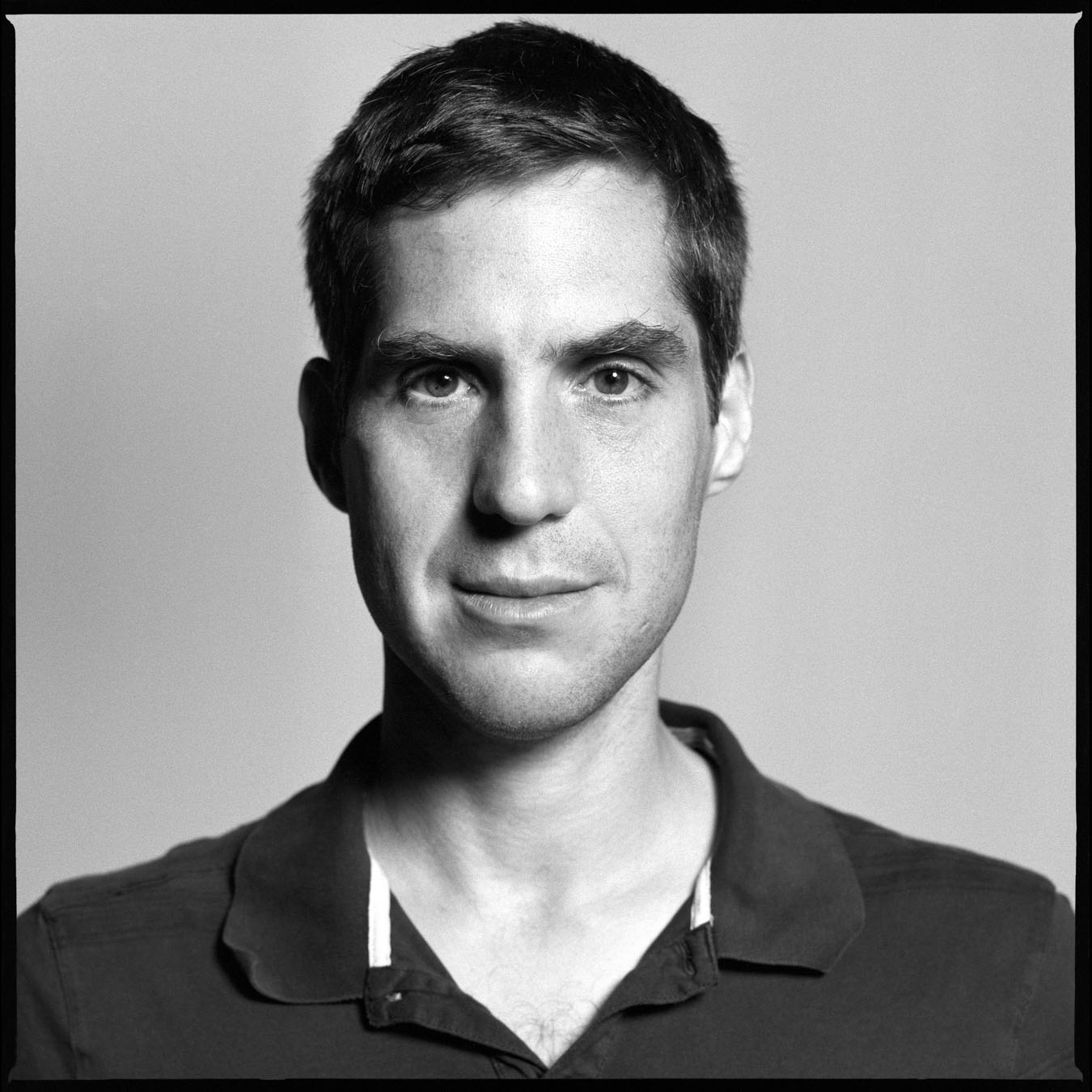# Laureates

### Jacob Lurie

Harvard University

#### 2015 Breakthrough Prize in Mathematics

For his work on the foundations of higher category theory and derived algebraic geometry; for the classification of fully extended topological quantum field theories; and for providing a moduli-theoretic interpretation of elliptic cohomology.

#### The Science

Jacob Lurie's research lies at the intersection of two mathematical disciplines: topology (the mathematical study of shapes) and algebraic geometry (the study of polynomial equations and their solutions). He is particularly known for his work on the theory of “derived” algebraic geometry, which uses ideas from topology to analyze redundant information in systems of polynomial equations.